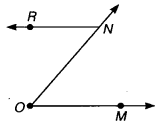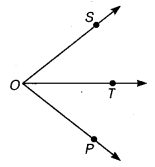# Class 6 Maths NCERT Solutions for Chapter 4 Basic Geometrical Ideas Ex – 4.3

## Basic Geometrical Ideas

Question 1.
Name the angles in the given figure.Solution:
The angles in the given figure are named as ∠ABC, ∠BCD, ∠CDA and ∠DAB.

Question 2.
In the given diagram, name the point(s)

(a) In the interior of ∠DOE
(b) In the exterior of ∠EOF
(c) On ∠EOF

Solution:
Clearly, from the given figure :

(a)
The point A lies in the interior of ∠DOE. .
(b) The points A and C lie in the exterior of ∠EOF.
(c) The points E, B, O and F lie on ∠EOF.

Question 3.
Draw rough diagrams of two angles such that they have

(a) One point in common
(b) Two points in common
(c) Three points in common
(d) Four points in common
(e) One ray in common

Solution:
(a) The diagram is shown as belowHere, ∠ROQ and ∠QOP have one point O in common.
(b) The diagram is shown as belowHere, ∠MON and ∠ONR have two points O and N in common.
(c) Drawing a diagram of two angles, such that they have three points in common, is not possible.
(d) Drawing a diagram of two angles, such that they have four points in common, is not possible.
(e) In the figure given below, ∠SOT and ∠POT have one ray$\overrightarrow { OT }$ in common.